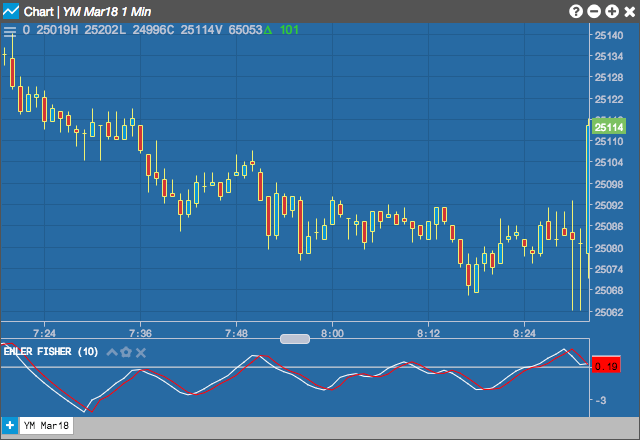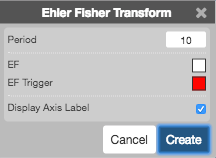Charts

# Ehler Fisher Transformation (EFT)

Ehler Fisher Transformation indicator tries to identify significant price reversals by normalizing prices over a user-specified number of periods. A reversal signal is suggested when the two lines cross.## Configuration Options• Period: Number of bars to use in the calculations.
• Color Selectors: Colors to use for graph elements.
• Display Axis Label: Whether to display the most recent value on the Y axis.

## Formula

$MidPoint = MP = \frac{High - Low)}{2}$

$Intermediate = I = 2 \times \frac{MP - LowestLow_{n-periods}}{HighestHigh_{n-periods}-LowestLow_{n-periods}}$

The intermediate term I is then smoothed by a 5-period exponential moving average (EMA) then transformed to a log form (fisher transform) before a final 3-period exponential moving average (EMA) smoothing:

$I_{smoothed} = EMA_{5-period}\;of\;I$

$EFT = EMA_{3-period}\;of\;log \left ( \frac{1+I_{smoothed}}{1-I_{smoothed}} \right )$